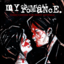MathematicsOpenStudy (anonymous):

Write the equation of a line parallel to the line 2x + 3y = 5 and passes through the point (9, -3) A. y equals negative two-thirds x plus 3 B. y equals negative two-thirds x plus five-thirds C. y = 2x – 21 D. y equals negative two-thirds x minus 9OpenStudy (anonymous):

A is rightOpenStudy (anonymous):

D.$y=-\frac{2 x}{3}-9$Plot attached. The red line is the answer.

Latest Questionshayhay6: Give an example of an equation of a line with an undefined slope. What type of li
1 minute ago 0 Replies 0 Medalshayhay6: What is the equation of a line that passes through the point (5, -3) and has a sl
5 minutes ago 1 Reply 0 Medalspiperkoebel: Does anyone know the coordinates of two points on the line y = u22122?
7 minutes ago 1 Reply 0 Medalslolokrat: anyone good with microeconomics that can help me find out where i went wrong on m
11 hours ago 0 Replies 0 MedalsAsleepAndUnafraid21: URGENT PLZ HELP NOW Part C: Find the distance from B to E and from P to E. Show your work.
12 hours ago 49 Replies 5 MedalsKachirikutoka: How many solutions does the following equation have? |3x + 12| = 18 a No solution
17 hours ago 1 Reply 1 MedalAsleepAndUnafraid21: In the figure below, segment DE is parallel to segment BC and segment EF is parallel to AB: The figure shows triangle ABC with segments DE and DF.
14 hours ago 11 Replies 0 MedalsAsleepAndUnafraid21: In the figure below, segment DE is parallel to segment BC and segment EF is parallel to AB: The figure shows triangle ABC with segments DE and DF.
18 hours ago 1 Reply 0 Medalslakeawilliams: What is the solution to StartFraction 5 over 6 EndFraction x minus one-third grea
19 hours ago 1 Reply 0 Medalskendall22: Identify the sentence that uses modifiers in the correct form. A. The food looked great but tasted awfully.
19 hours ago 1 Reply 0 Medals